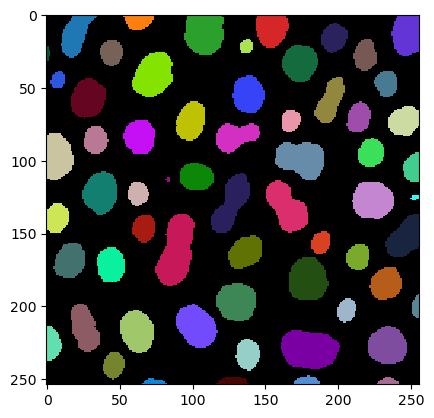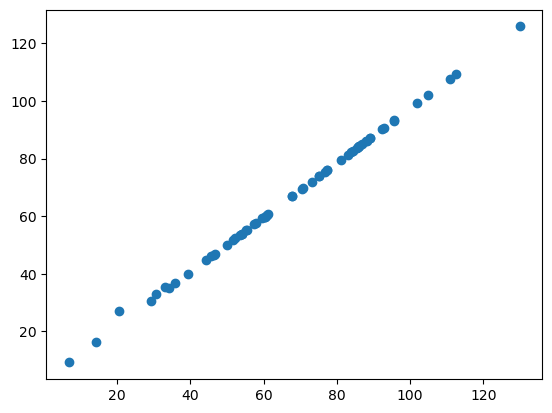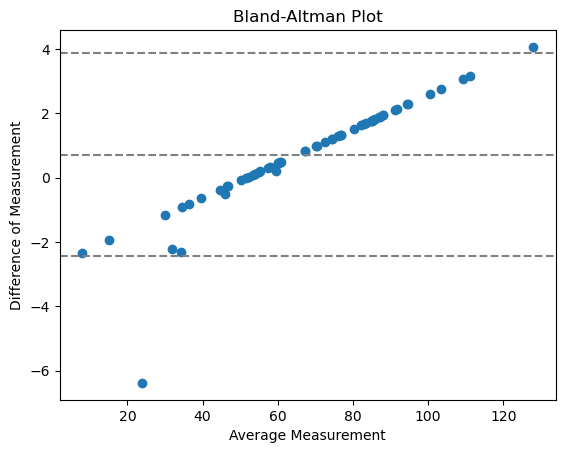# Comparison of measurements from different libraries#

We now compare the implementation of the measurement perimeter in napari-skimage-regionprops regionprops_table and napari-simpleitk-image-processing label_statistics.

import numpy as np
import pandas as pd
from pyclesperanto_prototype import imshow
from skimage import filters
from skimage import measure
import matplotlib.pyplot as plt
from napari_skimage_regionprops import regionprops_table
from skimage import filters
from napari_simpleitk_image_processing import label_statistics

C:\Users\maral\mambaforge\envs\feature_blogpost\lib\site-packages\morphometrics\measure\label.py:7: TqdmExperimentalWarning: Using tqdm.autonotebook.tqdm in notebook mode. Use tqdm.tqdm instead to force console mode (e.g. in jupyter console)
from tqdm.autonotebook import tqdm


Therefore, we need an image and a label image.

# load image

# denoising
blurred_image = filters.gaussian(image, sigma=1)

# binarization
threshold = filters.threshold_otsu(blurred_image)
thresholded_image = blurred_image >= threshold

# labeling
label_image = measure.label(thresholded_image)

# visualization
imshow(label_image, labels=True)We derive the perimeter measurements using the regionprops_table function

skimage_statistics = regionprops_table(image, label_image, perimeter = True)
skimage_statistics

label area bbox_area equivalent_diameter convex_area max_intensity mean_intensity min_intensity perimeter perimeter_crofton standard_deviation_intensity
0 1 429 750 23.371345 479 232.0 191.440559 128.0 89.012193 87.070368 29.793138
1 2 183 231 15.264430 190 224.0 179.846995 128.0 53.556349 53.456120 21.270534
2 3 658 756 28.944630 673 248.0 205.604863 120.0 95.698485 93.409370 29.392255
3 4 433 529 23.480049 445 248.0 217.515012 120.0 77.455844 76.114262 35.852345
4 5 472 551 24.514670 486 248.0 213.033898 128.0 83.798990 82.127941 28.741080
... ... ... ... ... ... ... ... ... ... ... ...
57 58 213 285 16.468152 221 224.0 184.525822 120.0 52.284271 52.250114 28.255467
58 59 79 108 10.029253 84 248.0 184.810127 128.0 39.313708 39.953250 33.739912
59 60 88 110 10.585135 92 216.0 182.727273 128.0 45.692388 46.196967 24.417173
60 61 52 75 8.136858 56 248.0 189.538462 128.0 30.692388 32.924135 37.867411
61 62 48 68 7.817640 53 224.0 173.833333 128.0 33.071068 35.375614 27.987596

62 rows × 11 columns

… and using the label_statistics function

simpleitk_statistics = label_statistics(image, label_image, size = False, intensity = False, perimeter = True)
simpleitk_statistics

label perimeter perimeter_on_border perimeter_on_border_ratio
0 1 87.070368 16.0 0.183759
1 2 53.456120 21.0 0.392846
2 3 93.409370 23.0 0.246228
3 4 76.114262 20.0 0.262763
4 5 82.127941 40.0 0.487045
... ... ... ... ...
57 58 52.250114 0.0 0.000000
58 59 39.953250 18.0 0.450527
59 60 46.196967 22.0 0.476222
60 61 32.924135 15.0 0.455593
61 62 35.375614 17.0 0.480557

62 rows × 4 columns

For this comparison, we are only interested in the column named perimeter. So we are selecting this column and convert the included measurements into a numpy array:

skimage_perimeter = np.asarray(skimage_statistics['perimeter'])
simpleitk_perimeter = np.asarray(simpleitk_statistics['perimeter'])


## Scatter plot#

If we now use matplotlib to plot the two perimeter measurements against each other, we receive the following scatter plot:

plt.plot(skimage_perimeter, simpleitk_perimeter, 'o')

[<matplotlib.lines.Line2D at 0x1912b551220>]If the two libraries would compute the perimeter in the same way, all of our datapoints would lie on a straight line. As you can see, they are not. This suggests that perimeter is implemented differently in napari-skimage-regionprops adn napari-simpleitk-image-processing.

## Bland-Altman plot#

The Bland-Altman plot helps to visualize the difference between measurements. We can compute a Bland-Altman plot for our two different perimeter implementations like this:

# compute mean, diff, md and sd
mean = (skimage_perimeter + simpleitk_perimeter) / 2
diff = skimage_perimeter - simpleitk_perimeter
md = np.mean(diff) # mean of difference
sd = np.std(diff, axis = 0) # standard deviation of difference

plt.plot(mean, diff, 'o')

plt.axhline(md,           color='gray', linestyle='--')
plt.axhline(md + 1.96*sd, color='gray', linestyle='--')
plt.axhline(md - 1.96*sd, color='gray', linestyle='--')

# add title and axes labels
plt.title('Bland-Altman Plot')
plt.xlabel('Average Measurement')
plt.ylabel('Difference of Measurement')

Text(0, 0.5, 'Difference of Measurement')With

• center line = mean difference between the methods

• two outer lines = confidence interval of agreement (CI)

The points do not go towards 0 which means it is not an agreement with random relative error, but systematic. This makes a lot of sense, because we are comparing here the implementation of a measurement.

# Exercise#

Use the functions regionprops_table and label_statistics to measure feret_diameter_max of your label image. Plot the Scatter plot and the Bland-Altman plot. Do you think feret_diameter_max is implemented differently in the two libraries?